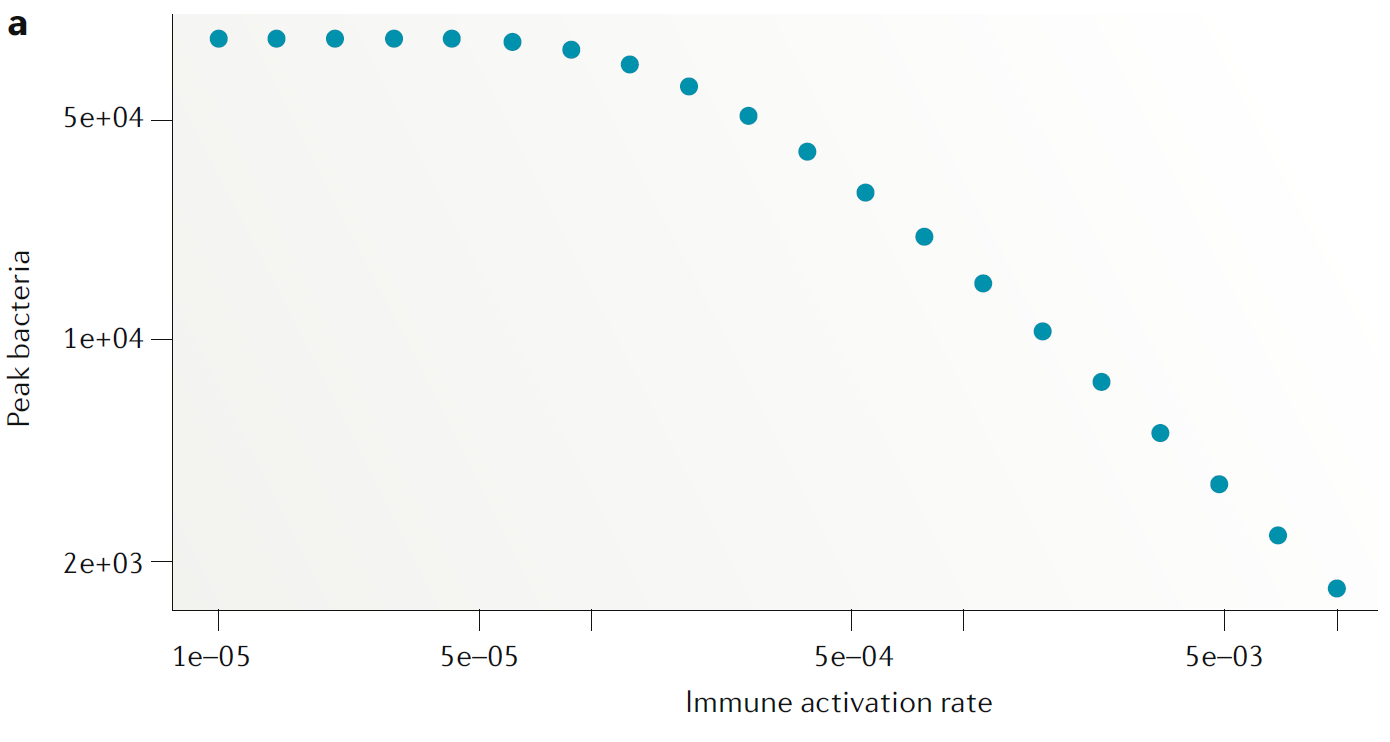2020-07-21 14:05:34

## Model uses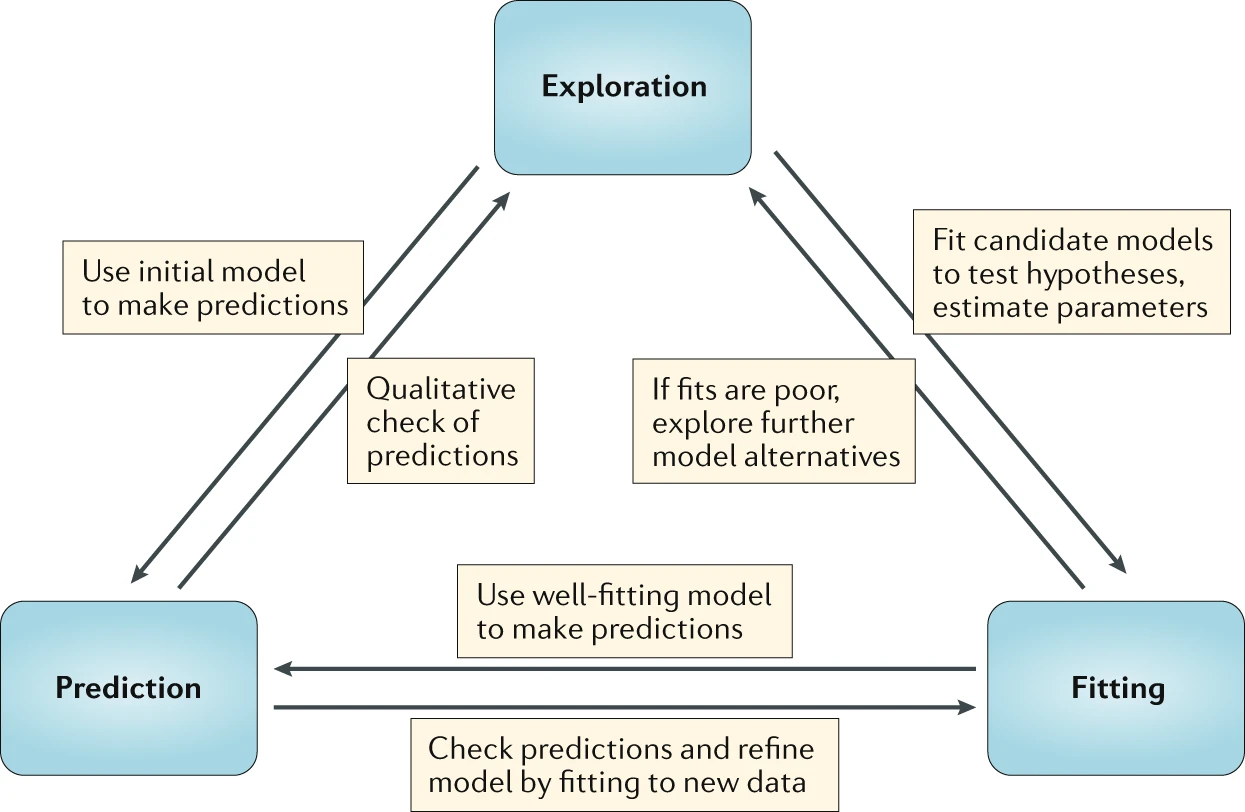## Model Exploration example

• Question: How dose the antigen dose for a killed (influenza) vaccine affect antibody levels post vaccination?
• Approach: Build a simple model and explore (after Handel et al 2018 PCB).

\begin{aligned} \dot V &= - d_V V - k_A AV \\ \dot F &= p_F - d_F F + \frac{V}{V+ h_V}g_F(F_{max}-F) \\ \dot B & = \frac{F V}{F V + h_F} g_B B \\ \dot A & = r_A B - d_A A - k_A A V \end{aligned} (This is a simpler version of the virus and immune response DSAIRM model.)

## Model Exploration example

Run model for different antigen doses ($$V_0$$).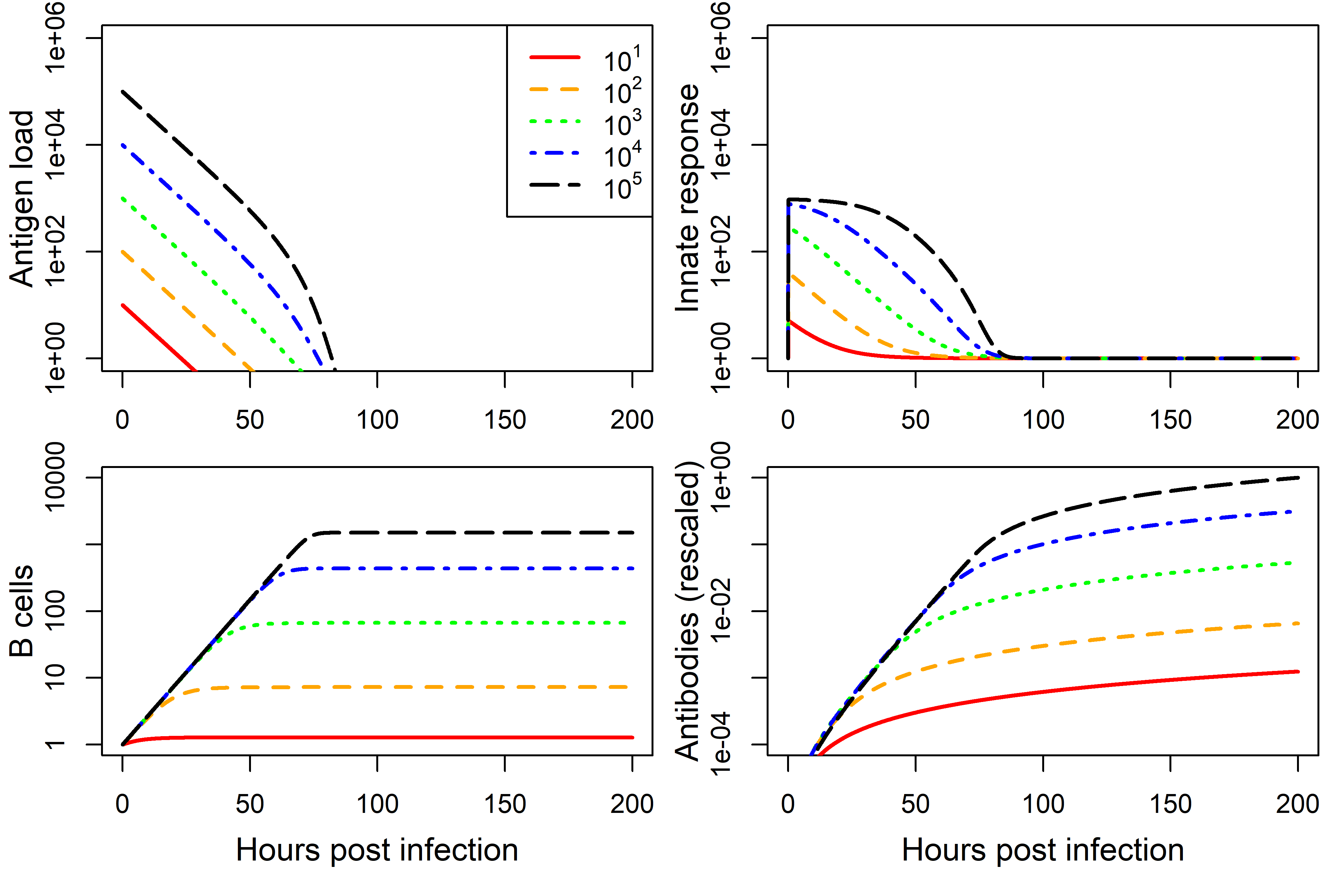## Model Exploration

• Looking at the dynamics (time-series) of a model can be useful.
• Often, we are not mainly interested in the time series, but instead some more specific quantity, e.g. total number of infected/pathogens, steady state values, etc.
• We usually want to to know how such outcome(s) of interest vary with some parameter(s).
• For our example, we want to know how antibody levels vary with vaccine dose, and how that affects protection from infection.
• What do we need to do to answer that question?

## Model Exploration

1. Choose some parameter values.
2. Run the simulation model.
3. Record quantities/outcomes of interest.
4. Choose another set of parameter values (usually we only vary one at a time).
5. Repeat steps 2-4 until you got all parameter-outcome pairs of interest.
6. Report (e.g. plot) your findings.

## Model Exploration

• Run model for different $$V_0$$, record antibodies $$A$$ at end of each simulation for each $$V_0$$.
• Use this equation to compute protection as a function of antibody level. $$P= 1 - \frac{1}{e^{k_1(\log(A)-k_2)}}$$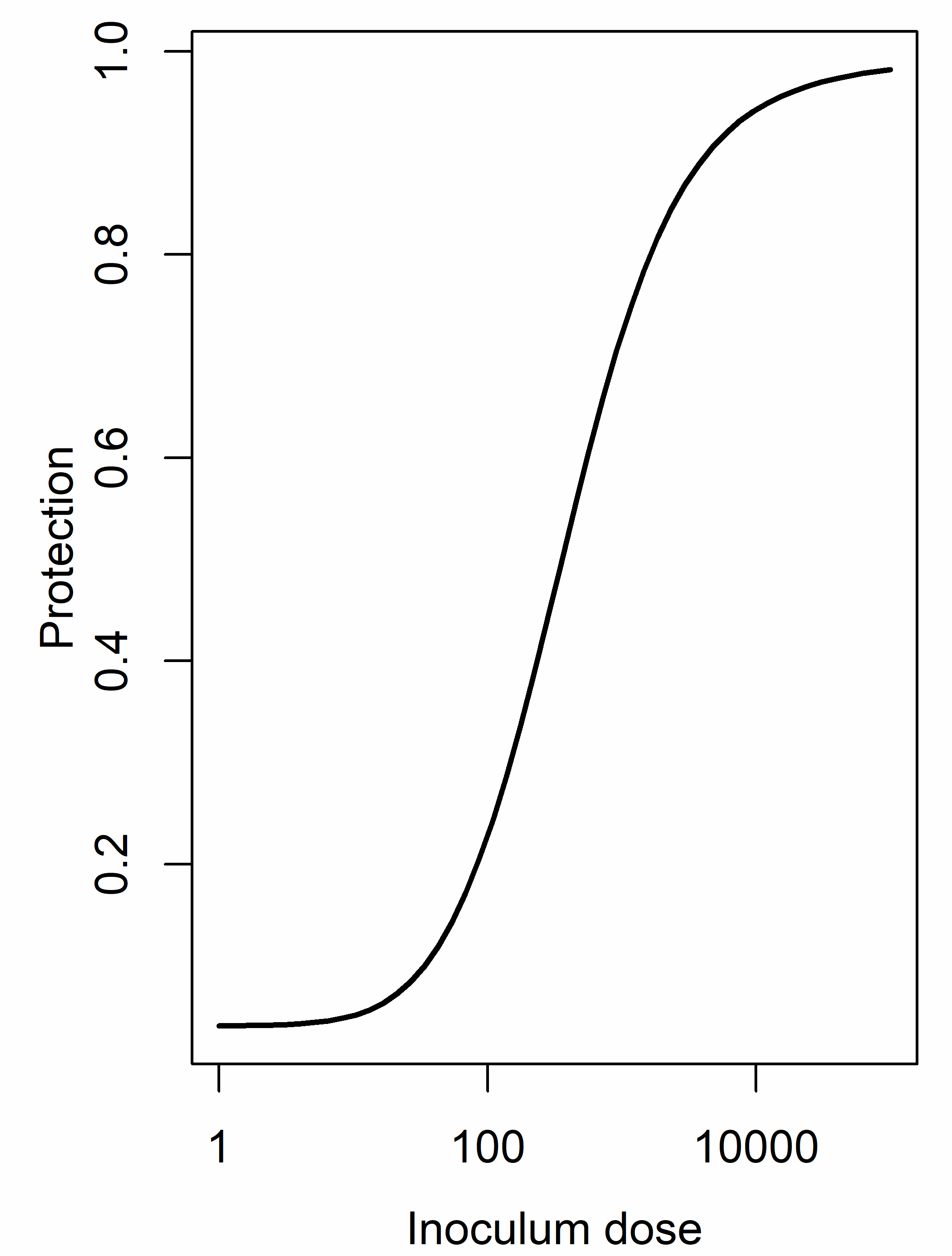## Model Exploration - Example 2

Virus fitness as function of virion binding ( $$k_+$$ ) and release ( $$k_-$$ ) rates. Handel et al (2014) Proc Royal Soc Interface.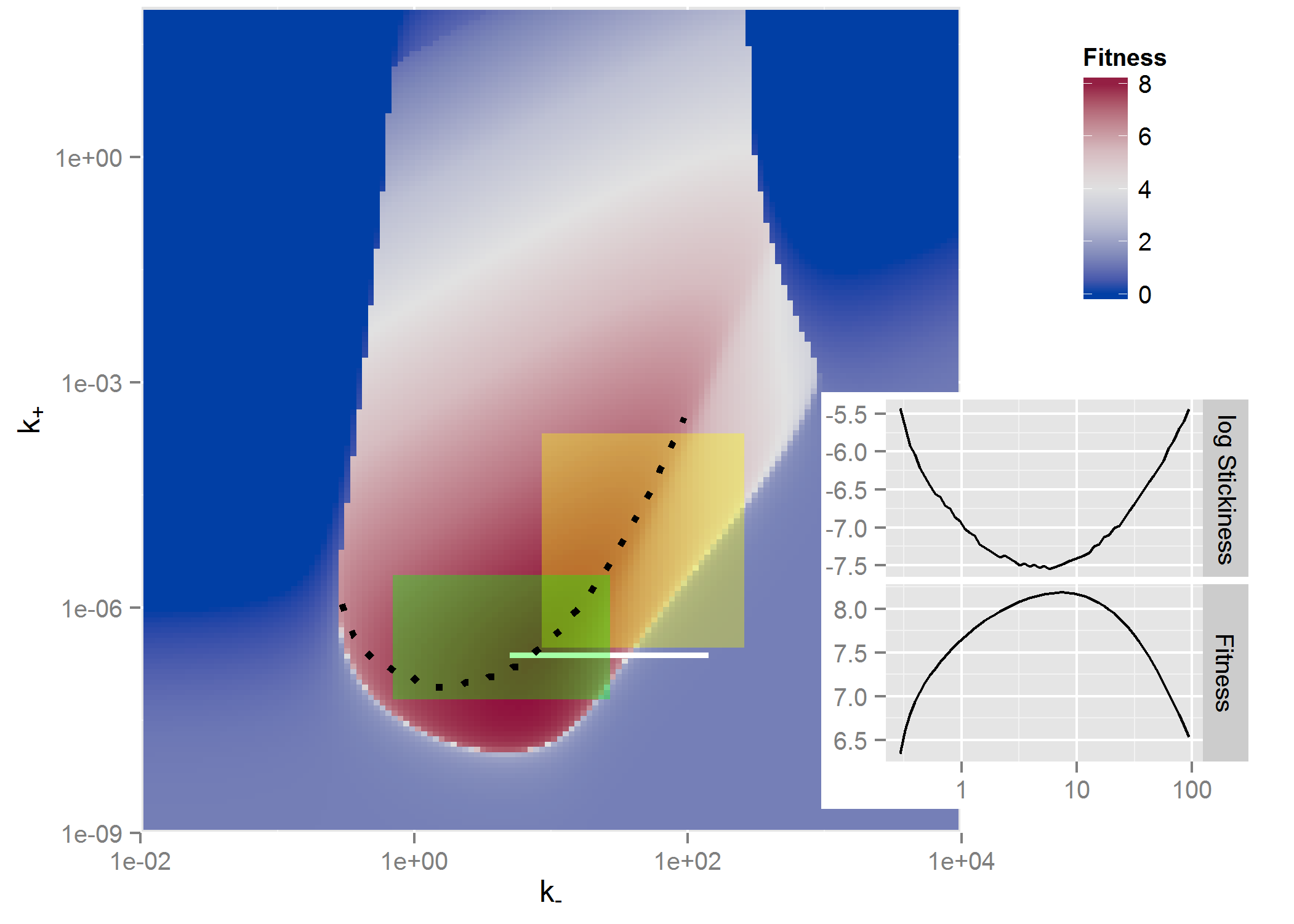## Exploration - summary

• If the system/question is very simple, we might not need a model.
• Interactions among pathogens and the immune response are often complex. If we know little about our system and its behavior, building and exploring simple models is often a useful first step.

## Exploration - practice

• We could do the model exploration by hand through the DSAIRM GUI.
• We could automate it by writing R code that loops over parameters and repeatedly calls the underlying model (see e.g. ‘Level 2’ in the package tutorial).
• The Model Exploration apps allows you to do such exploration graphically.

## Model-based predictions## Model Predictions/Virtual Experiments

• We saw how we can use models to explore how outcomes of interest change with parameters.
• Model exploration is often useful to gain general insights into a system early on.
• Once we built up our understanding and have a model that we think approximates reality reasonably well, we can potentially move on to making predictions and explore ‘what-if’ scenarios (virtual experiments).

## Prediction types

• Predictions can be of different types:
• Qualitative: Try to predict shape/direction of an outcome (similar to the ‘exploration’ model use).
• Semi-quantitative: Try to predict the approximate or relative size of an outcome.
• Quantitative: Try to predict (with confidence intervals) the magnitude of an outcome.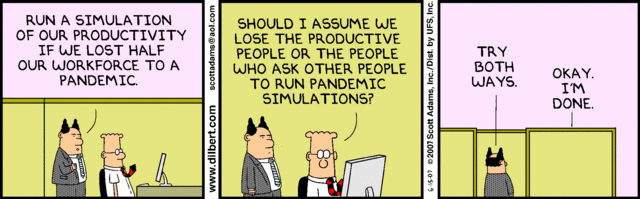dilbert.com

## Prediction example

Assume we think this model is a good approximation for a real system we are interested in. We want to predict the peak burden of bacteria if we were able to increase the induction of the immune response (parameter $$r$$), e.g. by giving a drug.

\begin{aligned} \dot{B} & = g B(1-\frac{B}{B_{max}}) - d_B B - kBI\\ \dot{I} & = r BI - d_I I \end{aligned}

## Prediction example

We’ll follow the same approach as for model exploration, the difference is that now we interpret the results as actual predictions instead of suggested findings that need to be further explored.